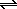# Suppose a 500.mL flask is filled with 1.1mol of NO2 1.6mol of CO and 0.90mol of...

###### Question:

Suppose a 500.mL flask is filled with 1.1mol of NO2 1.6mol of CO and 0.90mol of NO. The following reaction becomes possible:

NO2(g) + CO(g)NO(g) +CO2(g)

The equilibrium constant K for this reaction is 0.211 at the temperature of the flask.

Calculate the equilibrium molarity of NO. Round your answer to two decimal places.

#### Similar Solved Questions

##### < Question 22 of 25 > For each reaction, identify another quantity that is equal to...
< Question 22 of 25 > For each reaction, identify another quantity that is equal to A Hixn- CH,(9) — C(g) + 4H(g) CH,(g) +20,(g) – CO,(g) + 2H2O(1) O 4 x bond energy of C-H O enthalpy of combustion of CH, O enthalpy of formation of CO, (g) 0-4 x bond energy of C-H enthalpy of combu...
##### How does the acceleration of an object depend on the net force acting on it if the total mass is constant?
How does the acceleration of an object depend on the net force acting on it if the total mass is constant?...
##### Rotenone is a potent inhibitor of Complex I. Atpenin is a potent inhibitor of Complex II....
Rotenone is a potent inhibitor of Complex I. Atpenin is a potent inhibitor of Complex II. Which do you expect to inhibit the proton motive force-why? (5 pts)...
##### How do you solve c+7<=3?
How do you solve c+7<=3?...
##### On defined by the matrix answers as a comma separated list. Enter each vector in the...
on defined by the matrix answers as a comma separated list. Enter each vector in the form (Use r for any a ). User for any arbitrary 12 ( (xi, x2, Determine the kernel and range of the tr kertr) range(t) Show that dm kert)+dim range(T)dim domain) dim kertT)+ dim range()-dim domain(T)...
##### Balance the chemical equation for the following redox reaction under acidic aqueous conditions with the smallest...
Balance the chemical equation for the following redox reaction under acidic aqueous conditions with the smallest whole-number coefficients possible using the half-reaction method. What is the coefficient of Cl? Cl2(g)+S2O3 (aq) Cl(aq)+ SO2(aq)...